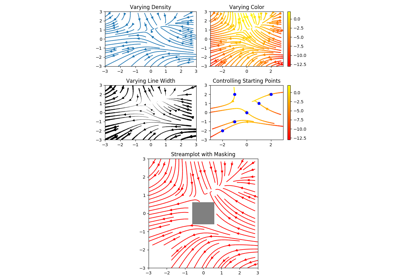# matplotlib.pyplot.streamplot¶

matplotlib.pyplot.streamplot(x, y, u, v, density=1, linewidth=None, color=None, cmap=None, norm=None, arrowsize=1, arrowstyle='-|>', minlength=0.1, transform=None, zorder=None, start_points=None, maxlength=4.0, integration_direction='both', *, data=None)[source]

Draw streamlines of a vector flow.

Parameters: x, y : 1d arrays An evenly spaced grid. u, v : 2d arrays x and y-velocities. Number of rows should match length of y, and the number of columns should match x. density : float or 2-tuple Controls the closeness of streamlines. When density = 1, the domain is divided into a 30x30 grid---density linearly scales this grid. Each cell in the grid can have, at most, one traversing streamline. For different densities in each direction, use [density_x, density_y]. linewidth : numeric or 2d array Vary linewidth when given a 2d array with the same shape as velocities. color : matplotlib color code, or 2d array Streamline color. When given an array with the same shape as velocities, color values are converted to colors using cmap. cmap : Colormap Colormap used to plot streamlines and arrows. Only necessary when using an array input for color. norm : Normalize Normalize object used to scale luminance data to 0, 1. If None, stretch (min, max) to (0, 1). Only necessary when color is an array. arrowsize : float Factor scale arrow size. arrowstyle : str Arrow style specification. See FancyArrowPatch. minlength : float Minimum length of streamline in axes coordinates. start_points : Nx2 array Coordinates of starting points for the streamlines. In data coordinates, the same as the x and y arrays. zorder : int Any number. maxlength : float Maximum length of streamline in axes coordinates. integration_direction : ['forward' | 'backward' | 'both'] Integrate the streamline in forward, backward or both directions. default is 'both'. stream_container : StreamplotSet Container object with attributes lines: matplotlib.collections.LineCollection of streamlines arrows: collection of matplotlib.patches.FancyArrowPatch objects representing arrows half-way along stream lines. This container will probably change in the future to allow changes to the colormap, alpha, etc. for both lines and arrows, but these changes should be backward compatible.

Notes

Note

In addition to the above described arguments, this function can take a data keyword argument. If such a data argument is given, the following arguments are replaced by data[<arg>]:

• All arguments with the following names: 'start_points', 'u', 'v', 'x', 'y'.

Objects passed as data must support item access (data[<arg>]) and membership test (<arg> in data).

## Examples using matplotlib.pyplot.streamplot¶Streamplot